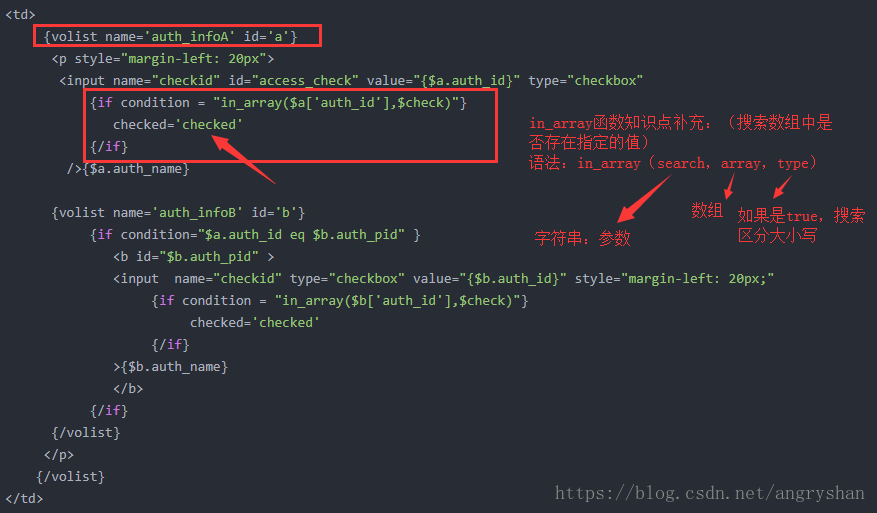# thinkPHP5 input接受数组 踩的坑

2022-02-27 11:06:51
1. `input('get.id/d');`
2. `input('post.name/s');`
3. `input('post.ids/a');`
4. `Request::instance()->get('id/d');`

ThinkPHP5.0版本默认的变量修饰符是`/s`，如果需要传入字符串之外的变量可以使用下面的修饰符，包括：

s强制转换为字符串类型
d强制转换为整型类型
b强制转换为布尔类型
a强制转换为数组类型
f强制转换为浮点类型

``````前台发送
\$.ajax({
method : 'post',
url : '{:url("Course/save_selected")}?course={\$course}',
data :  {
'noSelected' : nocheckedList,
'isSelected' : checkedList,
},
success : complete,
error:function(response){
//数据给后端后返回错误
console.log(response);//打印返回的信息
}
});

\$isSelected             = input('param.isSelected/a');//选中的数组   一维数组
\$noSelected             = input('param.noSelected/a');//未选中的数组 一维数组
````````````正常来说：
<input  name="checkid" type="checkbox" value="{\$b.auth_id}" style="margin-left: 20px;"
{if condition = "in_array(\$b['auth_id'],\$check)"}
checked='checked'
{/if}
>

<input type="checkbox" class="lesson_id" name="lesson_id[]" value="{{d[i].id}}"
{foreach name="Selected" item="vo"}
{{# if(d[i].id=={\$vo.lid}){ }}
checked
{{# } }}
{/foreach}
>

{foreach name="Selected" item="vo"}
{\$vo.lid}
{if condition="\$vo.lid == 7"}123123{/if}
{/foreach}